More digital channels are bringing greater connectivity and more data is bringing added complexity to organizations. All this can feel chaotic or like a fog of information warfare. As a result, the pace of disruption and data expansion require visual tools that accelerate data wrangling and modeling. To overcome complexity, [...]Photograph by Poussin Jean, license CC BY-SA 3.0, via Wikimedia Commons

The partition problem has many variations, but recently I encountered it as an interactive puzzle on a computer. (Try a similar game yourself!) The player is presented with an old-fashioned pan-balance scale and a set of objects of different weights. The challenge is to divide (or partition) the objects into two group. You put one group of weights on one side of the scale and the remaining group on the other side so that the scale balances.

Here's a canonical example of the partition problem for two groups. The weights of six items are X = {0.4, 1.0, 1.2, 1.7, 2.6, 2.7}. Divide the objects into two groups of three items so that each group contains half the weight, which is 4.8 for this example. Give it a try! I'll give a solution in the next section.

As is often the case, there are at least two ways to solve this problem: the brute-force approach and an optimization method that minimizes the difference in weights between the two groups. One advantage of the brute-force approach is that it is guaranteed to find all solutions. However, the brute-force method quickly becomes impractical as the number of items increases.

This article considers brute-force solutions. A more elegant solution will be discussed in a future article.

### Permutations: A brute-force approach

Let's assume that the problem specifies the number of items that should be placed in each group. One way to specify groups is to use a vector of ±1 values to encode the group to which each item belongs. For example, if there are six items, the vector c = {-1, -1, -1, +1, +1, +1} indicates that the first three items belong to one group and the last three items belong to the other group.

One way to solve the partition problem for two groups is to consider all permutations of the items. For example, Y = {0.4, 1.7, 2.7, 1.0, 1.2, 2.6} is a permutation of the six weights in the previous section. The vector c = {-1, -1, -1, +1, +1, +1} indicates that the items {0.4, 1.7, 2.7} belong to one group and the items {1.0, 1.2, 2.6} belong to the other group. Both groups have 4.8 units of weight, so Y is a solution to the partition problem.

Notice that the inner product Y`*c = 0 for this permutation. Because c is a vector of ±1 values, the inner product is the difference between the sum of weights in the first group and the sum of weights in the second group. An inner product of 0 means that the sums are the same in both groups.

### A program to generate all partitions

Let's see how you can use the ALLPERM function in SAS/IML to solve the two-group partition problem. Since the number of permutations grows very fast, let's use an example that contains only four items. The weights of the four items are X = {1.2, 1.7, 2.6, 3.1}. We want two items in each group, so define c = {-1, -1, 1, 1}. We search for a permutation Y = π(X), such that Y`*c = 0. The following SAS/IML program generates ALL permutations of the integers {1,2,3,4}. For each permutation, the sum of the first two weights is compared to the sum of the last two weights. The permutations for which Y`*c = 0 are solutions to the partition problem.

```proc iml; X = {1.2, 1.7, 2.6, 3.1}; c = { -1, -1, 1, 1}; /* k = 2 */   /* Brute Force Method 1: Generate all permutations of items */ N = nrow(X); P = allperm(N); /* all permutations of 1:N */ Y = shape( X[P], nrow(P), ncol(P) ); /* reshape to N x N! matrix */ z = Y * c; /* want to find z=0 (or could minimize z) */ idx = loc(abs(z) < 1E-8); /* indices where z=0 in finite precision */ Soln = Y[idx, ]; /* return all solutions; each row is solution */```

This program generates P, a matrix whose rows contain all permutations of four elements. The matrix Y is a matrix where each row is a permutation of the weights. Therefore, Y*c is the vector of all differences. When a difference is zero, the two groups contain the same weights. The following statements count how many solutions are found and print the first solution:

```numSolns = nrow(soln); s = soln[1,]; print numSolns, s[c={'L1' 'L2' 'R1' 'R2'}];```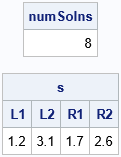There are a total of eight solutions. One solution is to put the weights {1.2, 3.1} in one group and the weights {1.7, 2.6} in the other group. What are the other solutions? For this set of weights, the other solutions are trivial variations of the first solution. The following statement prints all the solutions:

`print soln[c={'L1' 'L2' 'R1' 'R2'}];`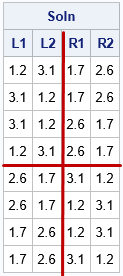I have augmented the output so that it is easier to see the structure. In the first four rows, the values {1.2, 3.1} are in the first group and the values {1.7, 2.6} are in the second group. In the last four rows, the values switch groups. Thus, this method, which is based on generating all permutations, generates a lot of solutions that are qualitatively the same, in practice.

### Combinations: Another brute-force approach

The all-permutation method generates N! possible partitions and, as we have seen, not all the partitions are qualitatively different. Thus, using all permutations is inefficient. A more efficient (but still brute-force) method is to use combinations instead of permutations. Combinations are essentially a sorted version of a permutation. The values {1, 2, 3}, {2, 1, 3}, and {3, 2, 1} are different permutations, whereas there is only one combination ({1, 2, 3}) that contains these three numbers. If there are six items and you want three items in each group, there are 6! = 720 permutations to consider, but only "6 choose 3" = 20 combinations.

The following SAS/IML function uses combinations to implement a brute-force solution of the two-group partition problem. The Partition2_Comb function takes a vector of item weights and a vector (nPart) that contains the number of items that you want in the first and second groups. If you want k items in the first group, the ALLCOMB function creates the complete set of combinations of k indices. The SETDIFF function computes the complementary set of indices. For example, if there are six items and {1, 4, 5} is a set of indices, then {2, 3, 6} is the complementary set of indices. After the various combinations are defined, the equation Y*c = 0 is used to find solutions, if any exist, where the first k elements of c are -1 and the last N-k elements are +1.

```/* Brute Force Method 2: Generate all combination of size k, N-k */ start Partition2_Comb(_x, k, tol=1e-8); x = colvec(_x); N = nrow(x); call sort(x); /* Optional: standardize the output */   c = j(k, 1, -1) // j(N-k, 1, 1); /* construct +/-1 vector */ L = allcomb(N, k); /* "N choose k" possible candidates in "left" group */ R = j(nrow(L), N-k, .); do i = 1 to nrow(L); R[i,] = setdif(1:N, L[i,]); /* complement indices in "right" group */ end; P = L || R; /* combine the left and right indices */   Y = shape( X[P], nrow(P) ); /* reshape X[P] into an N x (N choose k) matrix */ z = Y * c; /* want to find z=0 (or could minimize z) */ solnIdx = loc(abs(z) < tol); /* indices where z=0 in finite precision */ if ncol(solnIdx) = 0 then soln = j(1, N, .); /* no solution */ else soln = Y[solnIdx, ]; /* each row is solution */ return soln; /* return all solutions */ finish;   /* test the function on a set that has 11 items, partitioned into groups of 5 and 6 */ x = {0.8, 1.2, 1.3, 1.4, 1.6, 1.8, 1.9, 2.0, 2.2, 2.3, 2.5}; soln = Partition2_Comb(x, 5); numSolns = nrow(soln); print numSolns, soln[c=(('L1':'L5') || ('R1':'R6'))];```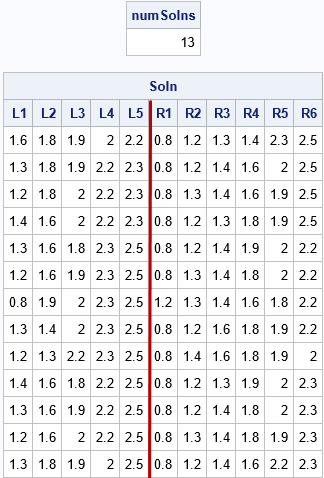The output shows 13 solutions for a set of 11 items that are partitioned into two groups, one with five items and the other with 11-5=6 items. For the solution, each partition has 9.5 units of weight.

### Summary

This article shows some SAS/IML programming techniques for using a brute-force method to solve the partition problem on N items. In the partition problem, you split items into two groups that have k and N-k items so that the weight of the items in each group is equal. This article introduced the solution in terms of all permutations of the items, but implemented a solution in terms of all combinations, which eliminates redundant orderings of the items.

In many applications, you don't need to find all solutions, you just need any solution. A subsequent article will discuss formulating the partition problem as an optimization that seeks to minimize the difference between the weights of the two groups.

The post The partition problem appeared first on The DO Loop.Many programmers are familiar with "short-circuit" evaluation in an IF-THEN statement. Short circuit means that a program does not evaluate the remainder of a logical expression if the value of the expression is already logically determined. The SAS DATA step supports short-circuiting for simple logical expressions in IF-THEN statements and WHERE clauses (Polzin 1994, p. 1579; Gilsen 1997). For example, in the following logical-AND expression, the condition for the variable Y does not need to be checked if the condition for variable X is true:

```data _null_; set A end=eof; if x>0 & y>0 then /* Y is evaluated only if X>0 */ count + 1; if eof then put count=; run;```

### Order the conditions in a logical statement by likelihood

SAS programmers can optimize their IF-THEN and WHERE clauses if they can estimate the probability of each separate condition in a logical expression:

• In a logical AND statement, put the least likely events first and the most likely events last. For example, suppose you want to find patients at a VA hospital that are male, over the age of 50, and have kidney cancer. You know that kidney cancer is a rare form of cancer. You also know that most patients at the VA hospital are male. To optimize a WHERE clause, you should put the least probable conditions first:
WHERE Disease="Kidney Cancer" & Age>50 & Sex="Male";
• In a logical OR statement, put the most likely events first and the least likely events last. For example, suppose you want to find all patients at a VA hospital that are either male, or over the age of 50, or have kidney cancer. To optimize a WHERE clause, you should use
WHERE Sex="Male" | Age>50 | Disease="Kidney Cancer";

The SAS documentation does not discuss the conditions for which a logical expression does or does not short circuit. Polzin (1994, p. 1579) points out that when you put function calls in the logical expression, SAS evaluates certain function calls that produce side effects. Common functions that have side effects include random number functions and user-defined functions (via PROC FCMP) that have output arguments. The LAG and DIF functions can also produce side effects, but it appears that expressions that involve the LAG and DIF functions are short-circuited. You can force a function evaluation by calling the function prior to an IF-THEN statement. You can use nested IF-THEN/ELSE statements to ensure that functions are not evaluated unless prior conditions are satisfied.

### Logical ligatures

The SAS/IML language does not support short-circuiting in IF-THEN statements, but it performs several similar optimizations that are designed to speed up your code execution. One optimization involves the ANY and ALL functions, which test whether any or all (respectively) elements of a matrix satisfy a logical condition. A common usage is to test whether a missing value appear anywhere in a vector, as shown in the following SAS/IML statement:

```bAny = any(y = .); /* TRUE if any element of Y is missing */ /* Equivalently, use bAll = all(y ^= .); */```

The SAS/IML language treats simple logical expressions like these as a single function call, not as two operations. I call this a logical ligature because two operations are combined into one. (A computer scientist might just call this a parser optimization.)

You might assume that the expression ANY(Y=.) is evaluated by using a two-step process. In the first step, the Boolean expression y=. is evaluated and the result is assigned to a temporary binary matrix, which is the same size as Y. In the second step, the temporary matrix is sent to the ANY function, which evaluates the binary matrix and returns TRUE if any element is nonzero. However, it turns out that SAS/IML does not use a temporary matrix. The SAS/IML parser recognizes that the expression inside the ANY function is a simple logical expression. The program can evaluate the function by looking at each element of Y and returning TRUE as soon it finds a missing value. In other words, it short-circuits the computation. If no value is missing, the expression evaluates to FALSE.

Short circuiting an operation can save considerable time. In the following SAS/IML program, the vector X contains 100 million elements, all equal to 1. The vector Y also contains 100 million elements, but the first element of the vector is a missing value. Consequently, the computation for Y is essentially instantaneous whereas the computation for X takes a tenth of a second:

```proc iml; numReps = 10; /* run computations 10 times and report average */ N = 1E8; /* create vector with 100 million elements */ x = j(N, 1, 1); /* all elements of x equal 1 */ y = x; y = .; /* the first element of x is missing */   /* the ALL and ANY functions short-circuit when the argument is a simple logical expression */ /* these function calls examine only the first elements */ t0 = time(); do i = 1 to numReps; bAny = any(y = .); /* TRUE for y */ bAll = all(y ^= .); /* TRUE for y */ end; t = (time() - t0) / numReps; print t[F=BEST6.];   /* these function calls must examine all elements */ t0 = time(); do i = 1 to numReps; bAny = any(x = .); bAll = all(x ^= .); end; t = (time() - t0) / numReps; print t[F=BEST6.];```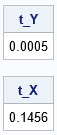Although the evaluation of X does not short circuit, it still uses the logical ligature to evaluate the expression. Consequently, the evaluation is much faster than the naive two-step process that is shown explicitly by the following statements, which require about 0.3 seconds and twice as much memory:

``` /* two-step process: slower */ b1 = (y=.); /* form the binary vector */ bAny = any(b1); /* TRUE for y */```

In summary, the SAS DATA step uses short-circuit evaluation in IF-THEN statements and WHERE clauses that use simple logical expressions. If the expression contains several subexpressions, you can optimize your program by estimating the probability that each subexpression is true. In the SAS/IML language, the ANY and ALL functions not only short circuit, but when their argument is a simple Boolean expression, the language treats the function call as a logical ligature and evaluates the call in an efficient manner that does not require any temporary memory.

Short circuits can be perplexing if you don't expect them. Equally confusing is expecting a statement to short circuit, but it doesn't. If you have a funny story about short-circuit operators, in any language, leave a comment.

The post Short-circuit evaluation and logical ligatures in SAS appeared first on The DO Loop.In SAS/IML programs, a common task is to write values in a matrix to a SAS data set. For some programs, the values you want to write are in a matrix and you use the CREATE FROM/APPEND FROM syntax to create the data set, as follows:

```proc iml; X = {1 2 3, 4 5 6, 7 8 9, 10 11 12}; create MyData from X[colname={'A' 'B' 'C'}]; /* create data set and variables */ append from X; /* write all rows of X */ close; /* close the data set */```

In other programs, the results are computed inside an iterative DO loop. If you can figure out how many observations are generated inside the loop, it is smart to allocate room for the results prior to the loop, assign the rows inside the loop, and then write to a data set after the loop.

However, sometimes you do not know in advance how many results will be generated inside a loop. Examples include certain kinds of simulations and algorithms that iterate until convergence. An example is shown in the following program. Each iteration of the loop generates a different number of rows, which are appended to the Z matrix. If you do not know in advance how many rows Z will eventually contain, you cannot allocate the Z matrix prior to the loop. Instead, a common technique is to use vertical concatenation to append each new result to the previous results, as follows:

```/* sometimes it is hard to determine in advance how many rows are in the final result */ free Z; do n = 1 to 4; k = n + floor(n/2); /* number of rows */ Y = j(k , 3, n); /* k x 3 matrix */ Z = Z // Y; /* vertical concatenation of results */ end; create MyData2 from Z[colname={'A' 'B' 'C'}]; /* create data set and variables */ append from Z; /* write all rows */ close; /* close the data set */```

Concatenation within a loop tends to be inefficient. As I like to say, "friends don't let friends concatenate results inside a loop!"

If your ultimate goal is to write the observations to a data set, you can write each sub-result to the data set from inside the DO loop! The APPEND FROM statement writes whatever data are in the specified matrix, and you can call the APPEND FROM statement multiple times. Each call will write the contents of the matrix to the open data set. You can update the matrix or even change the number of rows in the matrix. For example, the following program opens the data set prior to the DO loop, appends to the data set multiple times (each time with a different number of rows), and then closes the data set after the loop ends.

```/* alternative: create data set, write to it during the loop, then close it */ Z = {. . .}; /* tell CREATE stmt that data will contain three numerical variables */ create MyData3 from Z[colname={'A' 'B' 'C'}]; /* open before the loop. The TYPE of the variables are known. */ do n = 1 to 4; k = n + floor(n/2); /* number of rows */ Z = j(k , 3, n); /* k x 3 matrix */ append from Z; /* write each block of data */ end; close; /* close the data set */```

The following output shows the contents of the MyData3 data set, which is identical to the MyData2 data set: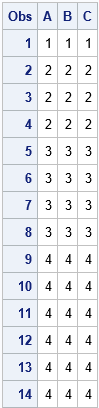Notice that the CREATE statement must know the number and type (numerical or character) of the data set variables so that it can set up the data set for writing. If you are writing character variables, you also need to specify the length of the variables. I typically use missing values to tell the CREATE statement the number and type of the variables. These values are not written to the data set. It is the APPEND statement that writes data.

I previously wrote about this technique in the article "Writing data in chunks," which was focused on writing large data set that might not fit into RAM. However, the same technique is useful for writing data when the total number of rows is not known until run time. I also use it when running simulations that generate multivariate data. This technique provides a way to write data from inside a DO loop and to avoid concatenating matrices within the loop.

The post Write to a SAS data set from inside a SAS/IML loop appeared first on The DO Loop.This article compares several ways to find the elements that are common to multiple sets. I test which method is the fastest in the SAS/IML language. However, all algorithms are intrinsically fast, which raises an important question: when is it worth the time and effort to optimize an algorithm?

The idea for this topic came from reading a blog post by 'mikefc' at the "Cool but Useless" blog about the relative performance of various ways to intersect vectors in R. Mikefc used vectors that contained between 10,000 and 100,000 elements and whose intersection was only a few dozen elements. In this article, I increase the sizes of the vectors by an order of magnitude and time the performance of the intersection function in SAS/IML. I also ensure that the sets share a large set of common elements so that the intersection is sizeable.

### The intersection of large sets

In general, finding the intersection between sets is a fast operation. In languages such as R, MATLAB, and SAS/IML, you can store the sets in vectors and use built-in functions to form the intersection. Because the intersection of two sets is always smaller than (or equal to) the size of the original sets, computing the "intersection of intersections" is usually faster than computing the intersection of the original (larger) sets.

The following SAS/IML program creates eight SAS/IML vectors that have between 200,000 and 550,000 elements. The elements in the vectors are positive integers, although they could also be character strings or non-integers. The vectors contain a large subset of common elements so that the intersection is sizeable. The vectors A, B, ..., H are created as follows:

```proc iml; call randseed(123); NIntersect = 5e4; common = sample(1:NIntersect, NIntersect, "replace"); /* elements common to all sets */   source = 1:1e6; /* elements are positive integers less than 1 million */ BaseN = 5e5; /* approx magnitude of vectors (sets) */ A = sample(source, BaseN, "replace") || common; /* include common elements */ B = sample(source, 0.9*BaseN, "replace") || common; C = sample(source, 0.8*BaseN, "replace") || common; D = sample(source, 0.7*BaseN, "replace") || common; E = sample(source, 0.6*BaseN, "replace") || common; F = sample(source, 0.5*BaseN, "replace") || common; G = sample(source, 0.4*BaseN, "replace") || common; H = sample(source, 0.3*BaseN, "replace") || common;```

The intersection of these vectors contains about 31,600 elements. You can implement the following methods that find the intersection of these vectors:

1. The XSECT function in SAS/IML accepts up to 15 arguments. You can therefore make a single call to find the intersection.
2. If you have dozens or hundreds of sets to intersect, you can loop over the sets and call the XSECT function multiple times. (Recall that the VALUE function enables you to loop over the names of the sets and access the vectors by names.) For example, you can let W1 be the intersection of the first two sets, let W2 be the intersection of W1 with the third set, and so forth. Because the size of the intersection is typically smaller than the size of the sets, later intersections require less time to compute than earlier intersections. (Although I only implement pairwise intersections, you could conceivably intersect more than two sets at a time.)
3. As 'mikefc' mentions, it is quicker to intersect small sets than to intersect large sets. Thus you can preprocess the names of the sets and sort them by size. This heuristic might make a difference when the set sizes vary greatly.

The following SAS/IML statements implement these three methods and compute the time required to intersect each:

```/* 1. one call to the built-in method */ t0 = time(); w = xsect(a,b,c,d,e,f,g,h); tBuiltin = time() - t0;   /* 2. loop over variable names and form pairwise intersections */ varName = "a":"h"; t0 = time(); w = value(varName); do i = 2 to ncol(varName); w = xsect(w, value(varName[i])); /* compute pairwise intersection */ end; tPairwise = time() - t0;   /* 3. Sort by size of sets, then loop */ varName = "a":"h"; t0 = time(); len = j(ncol(varName), 1); do i = 1 to ncol(varName); len[i] = ncol(value(varName[i])); /* number of elements in each set */ end; call sortndx(idx, len); /* sort smallest to largest */ sortName = varName[,idx]; w = value(sortName); do i = 2 to ncol(sortName); w = xsect(w, value(sortName[i])); /* compute pairwise intersection */ end; tSort = time() - t0;   print tBuiltin tPairwise tSort;```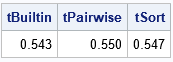For this example data, each method takes about 0.5 seconds to find the intersection of eight sets that contain a total of 3,000,000 elements. If you rerun the analysis, the times will vary by a few hundredths of a second, so the times are essentially equal. ('mikefc' also discusses a few other methods, including a "tally method." The tally method is fast, but it relies on the elements being positive integers.)

### Whether to optimize or not

There is a quote that I like: "In theory, there is no difference between theory and practice. But, in practice, there is." (Published by Walter J. Savitch, who claims to have overheard it at a computer science conference.) For the intersection problem, you can argue theoretically that pre-sorting by length is an inexpensive way to potentially obtain a boost in performance. However, in practice (for this example data), pre-sorting improves the performance by less than 1%. Furthermore, the absolute times for this operation are short, so saving a 1% of 0.5 seconds might not be worth the effort.

Even if you never compute the intersection of sets in SAS, there are two take-aways from this exercise:

• When you measure the performance of algorithms, use example data that is similar to the real data that you expect to encounter. The performance of this problem depends on the size of the sets, the number of sets, and the contents of the sets. The results that I show here are based on my choices for these parameters. Different parameters might yield different results.
• Always consider the total time for an algorithm before you start to optimize it. If your analysis takes 30 seconds, there is no need to optimize a step in the analysis that takes 0.5 seconds. No matter how well you optimize that step, it is not going to substantially shrink the total run time of your analysis.

The post The intersection of multiple sets appeared first on The DO Loop.A radial basis function is a scalar function that depends on the distance to some point, called the center point, c. One popular radial basis function is the Gaussian kernel φ(x; c) = exp(-||xc||2 / (2 σ2)), which uses the squared distance from a vector x to the center c to assign a weight. The weighted sum of Gaussian kernels, Σ wi φ(x; c) arises in many applications in statistics, including kernel density estimation, kernel smoothing, and machine learning algorithms such as support vector machines. It is therefore important to be able to efficiently evaluate a radial basis function and compute a weighted sum of several such kernel functions.

One of the many useful features of the SAS/IML language is its ability to compactly represent matrix and vector expressions. The expression ||xc|| looks like the distance between two vectors, but in the SAS/IML language the DISTANCE function can handle multiple sets of vectors:

• The DISTANCE function can compute the distance between two vectors of arbitrary dimensions. Thus when x and c are both d-dimensional row vectors, you can compute the distance by using r = DISTANCE(x, c). The result is a scalar distance.
• The DISTANCE function can compute the distance between multiple points and a center. Thus when x is an m x d matrix that contains m points, you can compute the m distances between the points and c by using r = DISTANCE(x, c). Again, the syntax is the same, but now r is an m x 1 vector of distances.
• The DISTANCE function in SAS/IML 14.3 can compute the distance between multiple points and multiple centers. Thus when x is an m x d matrix that contains m points and c is a p x d matrix that contains p centers, you can compute the m*p distances between the points and c by using r = DISTANCE(x, c). The syntax is the same, but now r is an m x p matrix of distances.

### A SAS/IML function that evaluates a Gaussian kernel function

The following SAS/IML statements define a Gaussian kernel function. Notice that the function is very compact! To test the function, define one center at C = (2.3, 3.2). Because SAS/IML is a matrix language, you can evaluate the Gaussian kernel on a grid of integer coordinates (x,y) where x is an integer in the range [1,5] and y is in the range [1,8]. Let Z be the matrix of the 40 ordered pairs. The following call evaluates the Gaussian kernel at the grid of points:

```proc iml; /* Radial basis function (Gaussian kernel). If z is m x d and c is n x d, this function returns the mxn matrix of values exp( -||z[i,] - c[j,]||**2 / (2*sigma**2) ) */ start GaussKernel(z, c, sigma=1); return exp( -distance(z,c)##2 / (2*sigma**2) ); finish;   /* test on small data: Z is an 5 x 8 grid and C = {2.3 3.2} */ xPts = 1:5; yPts = 1:8; Z = expandgrid(xPts, yPts); /* expand into (8*5) x 2 matrix */ C = {2.3 3.2}; /* the center */ phi = GaussKernel(Z, C); /* phi is 40 x 1 vector */   print Z phi; /* print in expanded form */ phi_Grid = shapecol(phi, ncol(yPts)); /* reshape into grid (optional) */ print phi_Grid[c=(char(xPts)) r=(char(yPts)) F=4.2];```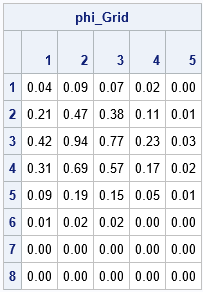The table shows the Gaussian kernel evaluated at the grid points. The columns represent the values at the X locations and the rows indicate the Y locations. The function is largest at the value (x,y)=(2,3) because (2,3) is the grid point closest to the center (2.3, 3.2). The largest value 0.94. Notice that the function is essentially zero at points that are more than 3 units from the center, which you would expect from a Gaussian distribution with σ = 1.

You can use the HEATMAPCONT subroutine to make a heat map of the function values. However, notice that in the matrix the rows increase in the downward direction, whereas in the usual Cartesian coordinate system the Y direction increases upward. Consequently, you need to reverse the rows and the Y-axis labels when you create a heat map:

```start PlotValues( v, xPts, yPts ); G = shapecol(v, ncol(yPts)); /* reshape vector into grid */ M = G[nrow(G):1, ]; /* flip Y axis (rows) */ yRev = yPts[, ncol(yPts):1]; /* reverse the Y-axis labels */ call heatmapcont(M) xvalues=xPts yValues=yRev; finish; run PlotValues(phi, xPts, yPts);```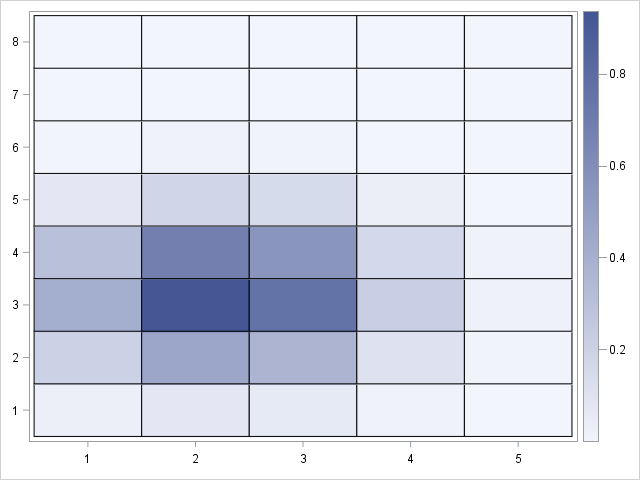### Sums of radial basis functionsOften the "centers" are the locations of some resource such as a warehouse, a hospital, or an ATM. Let's use the locations of 86 large US cities, which I used in a previous article about spatial data analysis. A graph of the locations of the cities is shown to the right. (Click to enlarge.) The locations are in a standardized coordinate system, so they do not directly correspond to longitudes and latitudes.

If there are multiple centers, the GaussKernel function returns a column for every center. Many applications require a weighted sum of the columns. You can achieve a weighted sum by using a matrix-vector product A*w, where w is a column vector of weights. If you want an unweighted sum, you can use the SAS/IML subscript reduction operator to sum across the columns: A[,+].

For example, the following statements evaluate the Gaussian kernel function at each value in a grid (the Z matrix) and for each of 86 cities (the C matrix). The result is a 3726 x 86 matrix of values. You can use the subscript reduction operator to sum the kernel evaluations over the cities, as shown:

```use BigCities; read all var {x y} into C; /* C = (x,y) locations of centers */ read all var "City"; close;   /* Z = a regular grid in (x,y) coordinates that contains the data */ XGridPts = round( do(-0.4, 0.4, 0.01), 0.001); YGridPts = round( do(-0.2, 0.25, 0.01), 0.001); Z = expandgrid( XGridPts, YGridPts ); /* 3,726 points on a 81x46 grid */   phi = GaussKernel(X, C, 0.025); /* use smaller bandwidth */ sumPhi = phi[,+]; /* for each grid point, add sum of kernel evaluations */```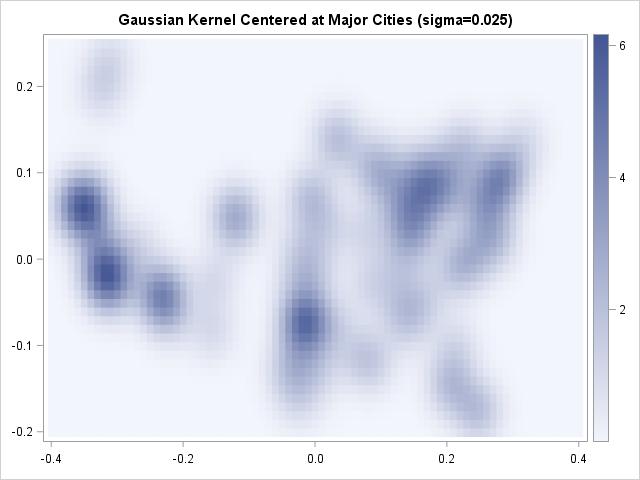The resulting heat map shows blobs centered at each large city in the data. Locations near isolated cities (such as Oklahoma City) are lighter in color than locations near multiple nearby cities (such as southern California and the New York area) because the image shows the superposition of the kernel functions. At points that are far from any large city, the sum of the Gaussian kernel functions is essentially zero.

In summary, if you work with algorithms that use radial basis functions such as Gaussian kernels, you can use the SAS/IML language to evaluate these functions. By using the matrix features of the language and the fact that the DISTANCE function supports matrices as arguments, you can quickly and efficiently evaluate weighted sums of these kernel functions.

The post Radial basis functions and Gaussian kernels in SAS appeared first on The DO Loop.When you implement a statistical algorithm in a vector-matrix language such as SAS/IML, R, or MATLAB, you should measure the performance of your implementation, which means that you should time how long a program takes to analyze data of varying sizes and characteristics. There are some general tips that can help you eliminate bottlenecks in your program so that your program is fast as lightning! In fact, it is a little bit frightening how quickly you can become an expert in timing.

The following general principles apply regardless of the language that you use to implement the algorithm:

1. Use simulation to construct test data of varying sizes. By simulating data, you can easily vary the size of the size while preserving distributional characteristics. For example, you might want to test an algorithm on data that are normally distributed and contain 25, 50, 75, and 100 thousand observations. (Each language has different techniques to simulate data efficiently. For SAS software, see Simulating Data with SAS.)
2. Vary the distribution of the test data. Concentrate mainly on how the algorithm performs on typical data, but also test examples of "best case" and "worst case" scenarios for which the algorithm might run very quickly or very slowly. If the performance is affected by other factors such as the proportion of outliers or missing values, then include those factors in the study.
3. If possible, construct timing tests that run between 0.1 and 1 seconds. This length is long enough to be reliably measured but short enough that you can run many tests. If you try to time very short intervals (less than a millisecond), you will discover that the operating system constantly performs unrelated background tasks that can interfere with your timings, which leads to noisy measurements. For ultra-short intervals (less than a microsecond), you can encounter an "uncertainty principle" phenomena in which the very act measuring the performance of an algorithm affects the performance that you are trying to measure.
4. To reduce timing noise, call the routine multiple times and report the mean or median. If the performance can vary greatly (±20% or more), report the distribution of times by using quantiles or by displaying a box plot.
5. Use a "burn-in" call to reduce the impact of overhead costs. The first time a routine is called, it might require the operating system to load DLLs or allocate a huge block of memory. When the routine is called a second time, the operating system might have cached the needed information. If so, subsequent calls could be considerably faster than the first.
6. Use a line plot or box plots to present the performance results, where the vertical axis represents time and the horizontal axis represents the size of the data. If the performance characteristics depend on other factors, you can represent those factors by overlaying multiple lines or by constructing a panel of graphs.

### Timing the performance of algorithms in SAS/IML

This article is motivated by an R programmer who was trying to find the fastest way (on average) to check whether a vector contains more than one unique value. The programmer was timing the performance of five different methods in R in hopes of finding the fastest. I will use the same example, but will examine only two SAS/IML methods:

• The ALL function in the SAS/IML language tests whether all elements of a vector are equal to some specified value. Thus the expression ALL(x = x) is true only when all elements of a vector are the same.
• The UNIQUE function in the SAS/IML language returns an ordered list of the distinct elements of a vector. Thus the expression (ncol(UNIQUE(x))=1) is true only when a vector containsone distinct value.

The ALL function should be fast to discard non-constant vectors because it a "short-circuiting" operation. That is, as soon as it detects two different values, it returns 0 (false). If you have a vector with 100,000 elements, the ALL function might only examine a small number of elements to determine that the vector is not constant. In contrast, the UNIQUE function should be relatively slow: it always examines all the elements, and it allocates memory to return a sorted list of all unique values.

The following program illustrates many of the best practices. It constructs random binary vectors that contain between 100,000 and 1 million elements. Most elements are 0, but there is a small probability that an element could be 1. Some of the vectors will contain a 1 near the beginning of the vector (the best case for the ALL function), others will contain a 1 near the end (the worst case for ALL).

```proc iml; /* TIP: "Burn-in" by calling each important function once before you start timing */ x = randfun(N, "Bernoulli", 1/N); /* Simulate data for size */ r = all(x = x); /* method 1: The ALL function */ r = (ncol(unique(x)) = 1); /* method 2: The UNIQUE function */ /* end burn-in */   sizes = do(2,10,2)*1e5; /* TIP: choose sizes so test runs in reasonable time */ NReps = 300; /* TIP: call functions multiple times */   TotalTime = j(ncol(sizes), 2); /* result matrix: for each size, save average time */ do j = 1 to ncol(sizes); /* TIP: use vectors of different sizes */ N = sizes[j]; x = j(N, 1); /* TRICK: estimate time to generate the random vectors; subtract that time later */ t0 = time(); do i = 1 to NReps; call randgen(x, "Bernoulli", 1/N); end; tRand = time() - t0;   /* Method 1: time for NReps calls */ t0 = time(); do i = 1 to NReps; call randgen(x, "Bernoulli", 1/N); /* TIP: simulate data for size */ r = all(x = x); /* Method 1: The ALL function */ end; TotalTime[j,1] = time() - t0 - tRand; /* subtract time to generate random numbers */   /* Method 2: time for NReps calls */ t0 = time(); do i = 1 to NReps; call randgen(x, "Bernoulli", 1/N); /* TIP: simulate data for size */ r = (ncol(unique(x)) = 1); /* Method 2: The UNIQUE function */ end; TotalTime[j,2] = time() - t0 - tRand; /* subtract time to generate random numbers */ end; AvgTime = TotalTime / NReps; /* compute average time per call */ print AvgTime[c={ALL UNIQUE} r=(putn(sizes,'comma9.')) format=6.4];   /* TIP: create a series plot that overlays both curves */ Size = Sizes` // Sizes`; /* convert from wide to long data */ Time = AvgTime[,1] // AvgTime[,2]; Group = j(ncol(sizes), 1, "ALL") // j(ncol(sizes), 1, "UNIQUE"); title; call series(Size, Time) group=Group grid={x y} option="curvelabel" label={"Size", "Average Time per Call"};```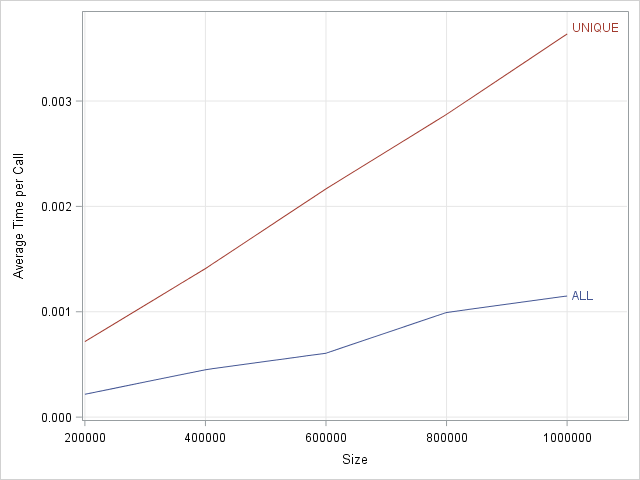The results are shown to the right. The graph shows the average time to determine whether the elements of a vector of size N are unique for N in the range [1e5, 1e6]. Notice that the graph shows the average time out of 300 different samples of data. As expected, the average time for the ALL function is less than the average time for the UNIQUE function. For the ALL function, some of the tests run almost instantaneously, whereas others require longer run times. The method that calls the UNIQUE function has less variation, although the variation is not shown in this graph.

In terms of absolute time, both methods require only a few milliseconds. In relative terms, however, the ALL method is much faster, and the relative difference increases as the size of the data increases.

Notice that the program demonstrates a useful trick. The ALL function runs much faster than the time required to generate a random vector with a million elements. Therefore the time required to generate a vector and determine whether it is constant is dominated by generating the data. To estimate ONLY the time spent by the ALL and UNIQUE functions, you can either pre-compute the data or you can estimate how long it takes to generate the data and subtract that estimate from the total time. Because this particular test requires generating 300 vectors with 1 million elements, pre-computing and storing the vectors would require a lot of RAM, therefore I used the estimation trick.

In conclusion, when you are timing an algorithm in a vector-matrix language, follow the best practices in this article. The most important tip is to call the method multiple times and plot the average (as I have done here) or plot the distribution of times (not shown). Plotting the average gives you an estimate for the expected time whereas plotting the distribution enables you to estimate upper and lower bounds on the times.

SAS/IML programmers can find additional examples of timing performance in the following articles:

The post 6 tips for timing the performance of algorithms appeared first on The DO Loop.As my colleague Margaret Crevar recently wrote, it is useful to know how long SAS programs take to run. Margaret and others have written about how to use the SAS FULLSTIMER option to monitor the performance of the SAS system. In fact, SAS distributes a macro that enables you to parse SAS logs to extract performance and timing information.

But for researchers who are developing custom algorithms in the SAS/IML language, there is a much simpler way to monitor performance. You can use the TIME function in Base SAS to find out (to within about 15 milliseconds) how long it takes for a SAS/IML function or set of statements to run. I use this function often to assess how an algorithm scales with the size of the input data. The TIME function returns the time of day, so if you call it twice and compute the difference in times, you get the time (in seconds) that elapsed between calls.

For example, suppose that you need to compute eigenvalues of a large symmetric matrix. You might be interested in knowing how the algorithm scales with the size of the (square) input matrix. The following SAS/IML program uses the SQRVECH function to create symmetric matrices of size 500, 1000, ..., 2500. For each matrix the TIME function is called just before and immediately after a call to the EIGVAL function, which computes the eigenvalues of the matrix. The elapsed time is plotted against the size of the matrix:

```proc iml; size = T(do(500, 2500, 250)); /* 500, 1000, ..., 2500 */ time = j(nrow(size), 1); /* allocate room for results */ call randseed(12345); do i = 1 to nrow(size); n = size[i]; r = j(n*(n+1)/2, 1); call randgen(r, "uniform"); /* generate random elements */ A = sqrvech(r); /* form symmetric matrix */   t0 = time(); /* save the current time */ val = eigval(A); /* put computation here */ time[i] = time() - t0; /* compute elapsed time */ end;   title "Time versus Matrix Size"; call series(size, time) grid={x y};```The line plot (click to enlarge) shows the timing of the eigenvalue computation on square matrices of varying sizes. The computation is very fast when the matrices are less than 1000 x 1000, but takes longer as the matrix grows. For a 2500 x 2500 matrix, the computation takes about 15 seconds.

You can also use the TIME function to compare the performance of two or more different algorithms. For example, you can compare the performance of solving linear systems to help you write efficient programs.

You can also use this technique to time how long SAS procedures take to run: You can use the SUBMIT/ENDSUBMIT statements to call any SAS procedure, which means you can "drive" the performance analysis from the SAS/IML language. This technique is much easier than parsing SAS logs!

Incidentally, the distribution of the eigenvalues for a matrix with random elements that are drawn from a given distribution is a fascinating topic that is part of "random matrix theory." For a peek into this beautiful mathematical topic, see the article "The curious case of random eigenvalues", which discusses the symmetric matrices that I used in today's article. For unsymmetric matrices, the eigenvalues can be complex, and the distribution of the eigenvalues in the complex plane makes beautiful images.

For more details about timing computations and assessing the performance of algorithms, see Chapter 15 of Statistical Programming with SAS/IML Software.

The post Compare the performance of algorithms in SAS appeared first on The DO Loop.Imagine that you have one million rows of numerical data and you want to determine if a particular "target" value occurs. How might you find where the value occurs?

For univariate data, this is an easy problem. In the SAS DATA step you can use a WHERE clause or a subsetting IF statement to find the rows that contain the target value. In the SAS/IML language you can use the LOC function to find the rows.

For multivariate data, you can use a similar approach, except the code become messier. For example, suppose that you have a data set that contains five numeric variables and you want to test whether the 5-tuple (1, 2, 3, 4, 5) appears in the data. In the DATA step you might write the following statement:

`if x1=1 & x2=2 & x3=3 & x4=4 & x5=5 then ...;`

A general mathematical principle is that a "target problem" is equivalent to a root-finding problem. The standard mathematical trick is to subtract the target value and then find zeros of the resulting set of numbers. This trick provides the basis for writing a general SAS/IML programs that is vectorized and that can handle an arbitrary number of variables. The following statements generate a matrix that has one million rows and five columns. Each element is an integer 0 through 9.

```proc iml; call randseed(123); x = floor( 10*randfun({1e6 5}, "Uniform") ); /* matrix of values 0-9 */```

Suppose that you want to find whether there is a row that matches the target value {1 2 3 4 5}. The following statements subtract the target value from the data and then find all rows for which the difference is the zero vector:

```target = {1 2 3 4 5}; y = x - target; /* match target ==> y={0 0...0} */ count = (y=0)[,+]; /* count number of 0s in each row */ idx = loc(count=ncol(target)); /* rows that match target */ if ncol(idx)>0 then print "Target pattern found in " (ncol(idx)) " rows"; else print "Target pattern not found";```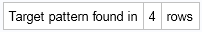The variable idx contains the row numbers for which the pattern occurs. For this random integer matrix, the target pattern appeared four times. Other random matrices might have six, 12, or 15 rows that match the target. It is easy to show that in a random matrix with one million rows, the target value is expected to appear 10 times.

The example in this article shows how to search a numerical matrix for rows that have a particular value. For character matrices, you can use the ROWCATC function in SAS/IML to concatenate the column values into a single vector of strings. You can then use the LOC function to find the rows that match the pattern.

tags: Data Analysis, EfficiencyEvaluating a cumulative distribution function (CDF) can be an expensive operation. Each time you evaluate the CDF for a continuous probability distribution, the software has to perform a numerical integration. (Recall that the CDF at a point x is the integral under the probability density function (PDF) where x is the upper limit of integration. I am assuming that the PDF does not have a closed-form antiderivative.) For example, the following statements compute and graph the CDF for the standard lognormal distribution at 121 points in the domain [0,6]. To create the graph, the software has to compute 121 numerical integrations.

```proc iml; x = do(0, 6, 0.05); CDF = cdf("Lognormal", x); title "Standard Lognormal CDF"; call series(x, CDF) grid={x y};```

### An integral is an accumulation of slices

In contrast, evaluating the PDF is relatively cheap: you only need to evaluate the density function at a series of points. No integrals reqired. This suggests a clever little approximation: Instead of calling the CDF function many times, call the PDF function and use the cumulative sum function (CUSUM) to add together the areas of many slices of the density. For example, it is simple to modify the trapezoidal rule of integration to return a cumulative sum of the trapezoidal areas that approximate the area under a curve, as follows:

```start CumTrapIntegral(x,y); N = nrow(colvec(x)); dx = x[2:N] - x[1:N-1]; meanY = ( y[2:N] + y[1:N-1] )/2; return( 0 // cusum(dx # meanY) ); finish;```

With this function you can approximate the (expensive) CDF by using a series of (cheap) PDF evaluations, as follows:

```pdf = pdf("Lognormal", x); /* evaluate PDF */ CDFApprox = CumTrapIntegral(x, pdf); /* cumulative sums of trapezoidal areas */```

How good is the approximation to the CDF? You can plot the difference between the values that were computed by the CDF function and the values that were computed by using the trapeoidal areas:

```Difference = CDF - CDFApprox`; title "Difference between Lognormal CDF and Aproximate CDF"; call Series(x, Difference) grid={x y};```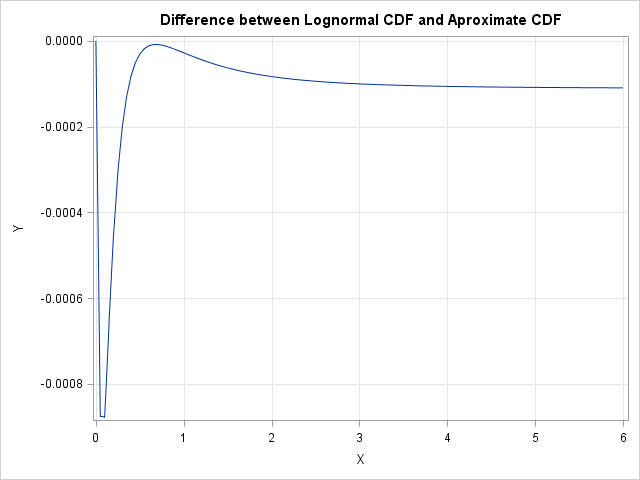The graph shows that for the lognormal distribution evaluated on [0,6], the approximate CDF differs by less than 0.001. For most values of x, CDF(x) differs from the cumulative trapezoidal approximation by less than 0.0001. This result uses PDF values with a step size of 0.05. In general, smaller step sizes lead to smaller errors, and the error for the trapezoidal rule is proportional to the square of the step size.

The lognormal distribution is exactly zero for x < 0, which means that the integral from minus infinity to 0 is zero. However, for distributions that are defined on the whole real line, you need to make a small modification to the algorithm. Nanmely, if you are approximating a cumulative distribution on the interal [a, b], you need to add the CDF value at a to the values returned by the CumTrapIntegral function. For example, the following statements compare the values of the standard normal CDF on [-3, 3] with the cumulative trapezoidal sums:

```/* for distributions on [-infinity, x], correct by adding constant */ x = do(-3, 3, 0.05); CDF = cdf("Normal", x); /* gold standard */ pdf = pdf("Normal", x); CDFApprox = CumTrapIntegral(x, pdf); /* cumulative sums */ CDFAdd = cdf("Normal", -3); /* integral on (-infinity, -3) */ CDFApprox = CDFAdd + CDFApprox`; /* trapezoidal approximation */   Difference = CDF - CDFApprox; title "Difference between Normal CDF and Aproximate CDF"; call Series(x, Difference) grid={x y};```

The graph shows that the approximation is within ±5e-5 of the values from the CDF function.

### When should you use this approximation?

You might be asking the same question that my former math students would ask: "When will I ever use this stuff?" One answer is to use the approximation when you are computing the CDF for a distribution that is not supported by the SAS CDF function. You can implement the CDF by using the QUAD subroutine, but you can use the method in this post when you only need a quick-and-dirty approximation to the CDF.

In my next blog post I will show how to graph the PDF and CDF for a distribution that is not built into SAS.